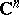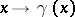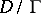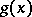# Automorphic form

(diff) ← Older revision | Latest revision (diff) | Newer revision → (diff)

A meromorphic function on a bounded domainof the complex spacethat, for some discrete group of transformationsoperating on this domain, satisfies an equation:Hereis the Jacobian of the mappingandis an integer known as the weight of the automorphic form. If the groupacts fixed-point free, then automorphic forms define differential forms on the quotient spaceand vice versa. Automorphic forms may be used in the construction of non-trivial automorphic functions (cf. Automorphic function). It has been shown that ifis a function that is holomorphic and bounded on a domain, then the seriesconverges for large values of, thus representing a non-trivial automorphic function of weight. These series are called Poincaré theta-series.

The classical definition of automorphic forms, given above, has recently served as the starting point of a far-reaching generalization in the theory of discrete subgroups of Lie groups and adèle groups.

How to Cite This Entry:
Automorphic form. Encyclopedia of Mathematics. URL: http://encyclopediaofmath.org/index.php?title=Automorphic_form&oldid=13843
This article was adapted from an original article by A.N. Parshin (originator), which appeared in Encyclopedia of Mathematics - ISBN 1402006098. See original article